SSC CGL Previous Year Questions: Algebra- 2

# SSC CGL Previous Year Questions: Algebra- 2 - SSC CGL

Test Description

## 40 Questions MCQ Test SSC CGL (Tier - 1) - Previous Year Papers (Topic Wise) - SSC CGL Previous Year Questions: Algebra- 2

SSC CGL Previous Year Questions: Algebra- 2 for SSC CGL 2023 is part of SSC CGL (Tier - 1) - Previous Year Papers (Topic Wise) preparation. The SSC CGL Previous Year Questions: Algebra- 2 questions and answers have been prepared according to the SSC CGL exam syllabus.The SSC CGL Previous Year Questions: Algebra- 2 MCQs are made for SSC CGL 2023 Exam. Find important definitions, questions, notes, meanings, examples, exercises, MCQs and online tests for SSC CGL Previous Year Questions: Algebra- 2 below.
Solutions of SSC CGL Previous Year Questions: Algebra- 2 questions in English are available as part of our SSC CGL (Tier - 1) - Previous Year Papers (Topic Wise) for SSC CGL & SSC CGL Previous Year Questions: Algebra- 2 solutions in Hindi for SSC CGL (Tier - 1) - Previous Year Papers (Topic Wise) course. Download more important topics, notes, lectures and mock test series for SSC CGL Exam by signing up for free. Attempt SSC CGL Previous Year Questions: Algebra- 2 | 40 questions in 40 minutes | Mock test for SSC CGL preparation | Free important questions MCQ to study SSC CGL (Tier - 1) - Previous Year Papers (Topic Wise) for SSC CGL Exam | Download free PDF with solutions
 1 Crore+ students have signed up on EduRev. Have you?
SSC CGL Previous Year Questions: Algebra- 2 - Question 1

### If 3x – 8(2 – x) = –19, then the value of x is       (SSC CHSL 2017)

Detailed Solution for SSC CGL Previous Year Questions: Algebra- 2 - Question 1

3x – 8 (2 – x) = –19
3x – 16 + 8x = – 19
11x = – 3
∴ x = (-3)/11

SSC CGL Previous Year Questions: Algebra- 2 - Question 2

### If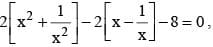then what two values of x - (1/x)?       (SSC CGL 2017)

SSC CGL Previous Year Questions: Algebra- 2 - Question 3

### What is the value of x in the equation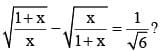(SSC CGL 2017)

Detailed Solution for SSC CGL Previous Year Questions: Algebra- 2 - Question 3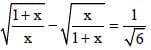By squaring both sides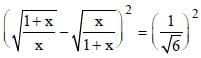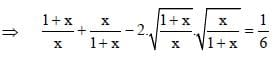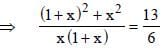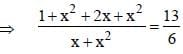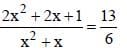⇒ 13x2 + 13x = 12x2 + 12x + 6
⇒ x2 + 3x – 2x – 6 = 0
⇒ x (x + 3) – 2 (x + 3) = 0
⇒ (x – 2) (x + 3) = 0
⇒ x – 2 = 0 ∴ x = 2
⇒ x + 3 = 0 ∴ x = – 3

SSC CGL Previous Year Questions: Algebra- 2 - Question 4

If x + (1/x) = 17, then what is the value of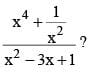(SSC CGL 2017)

Detailed Solution for SSC CGL Previous Year Questions: Algebra- 2 - Question 4

Here,
x + (1/x) = 17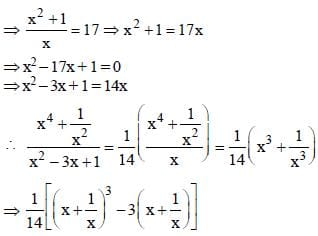= 1/14 (4913-3x17)
⇒ (1/14) x 4862 = 2431/7

SSC CGL Previous Year Questions: Algebra- 2 - Question 5

What is the value of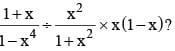(SSC CGL 2017)

Detailed Solution for SSC CGL Previous Year Questions: Algebra- 2 - Question 5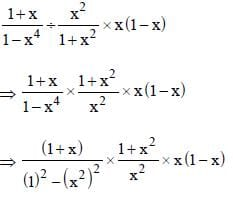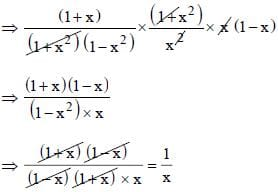SSC CGL Previous Year Questions: Algebra- 2 - Question 6

If p/q = r/s = t/u = √5, then what is the value of [(3p2 + 4r2 +  5t2) / (3q2 + 4s2 + 5u2)]?       (SSC CGL 2017)

Detailed Solution for SSC CGL Previous Year Questions: Algebra- 2 - Question 6

Here,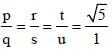then,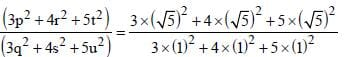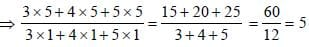SSC CGL Previous Year Questions: Algebra- 2 - Question 7

If x – y – √18 = – 1 and x + y – 3√2 = 1, then what is the value of 12xy (x2 – y2)?       (SSC CGL 2017)

Detailed Solution for SSC CGL Previous Year Questions: Algebra- 2 - Question 7

According to question,
x - y - √18 = -1 ...(i)
x + y - 3√2 = 1 ...(ii)
From equation (i) and (ii),
We get,
x = 3√2 and y = 1
then,
12xy (x2 – y2)
⇒ 12x3√2 x1[(3√2)2 - (1)2 ]
⇒ 36√2 x 17 = 612√2

SSC CGL Previous Year Questions: Algebra- 2 - Question 8

If (x – y) = 7, th en wh at is the value of (x – 15)3 – (y – 8)3?      (SSC CGL 2017)

Detailed Solution for SSC CGL Previous Year Questions: Algebra- 2 - Question 8

Here,
(x – y) = 7
then,
∴ a3 – b3 = (a – b) (a2 + ab + b2)
(x – 15)3 – (y – 8)3 = ?
⇒ (x – 15 – y + 8) [(x –15)2 + (x – 15) (y – 8) + (y – 8)2]
⇒ (x – y – 7)[ (x – 15)2 + (x – 15) (y – 8) + (y – 8)2]
⇒ (7 – 7) [(x – 15)2 + (x – 15) (y – 8) + (y – 8)2]
⇒ 0 × [(x – 15)2 + (x – 15) (y – 8) + (y – 8)2]
⇒ 0

SSC CGL Previous Year Questions: Algebra- 2 - Question 9

If (x – 2) an d (x + 3 ) are th e factor s of the equat ion x2 + k1x + k2 = 0, then what are the values of k1 and k2?      (SSC CGL 2017)

Detailed Solution for SSC CGL Previous Year Questions: Algebra- 2 - Question 9

∵ If x – 2 = 0
∴ x = 2
then,
x2 + k1x + k2 = 0
(2)2 + k1 × 2 + k2 = 0
2k1 + k2 = – 4 ...(i)
If x + 3 = 0
∴ x = –3
then,
x2 + k1x + k2 = 0
(–3)2 + k1x – 3 + k2 = 0
∴ 3k1 – k2 = 9 ...(ii)
From equation (i) and (ii),
we get k 1 = 1 and k2 = – 6

SSC CGL Previous Year Questions: Algebra- 2 - Question 10

If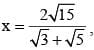then what is the value of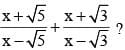(SSC CGL 2017)

SSC CGL Previous Year Questions: Algebra- 2 - Question 11

If a + b + c = 27, then what is the value of (a – 7)3 + (b – 9)3 + (c – 11)3 – 3(a – 7) (b – 9) (c – 11)?        (SSC CGL 2017)

Detailed Solution for SSC CGL Previous Year Questions: Algebra- 2 - Question 11

According to question,
a + b + c = 27
(a – 7) + (b – 9) + (c – 11) = 27 – 7 – 9 – 11
(a – 7) + (b – 9) + (c – 11) = 0
∴ a + b + c = 0
then
a3 + b3 + c3 – 3abc = 0

SSC CGL Previous Year Questions: Algebra- 2 - Question 12

If x = 6+ 2√6, then what is the value of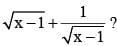(SSC CGL 2017)

Detailed Solution for SSC CGL Previous Year Questions: Algebra- 2 - Question 12

x = 6 + 2√6
Subtraction by 1 in both side.
x -1 = 6 + 2√6 - 1
x -1 = 5 + 2√6 ⇒ 3 + 2√6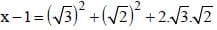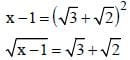Now,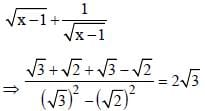SSC CGL Previous Year Questions: Algebra- 2 - Question 13

If x + (1/x) = 2, then what is the value of x64 + x121?        (SSC CGL 2017)

Detailed Solution for SSC CGL Previous Year Questions: Algebra- 2 - Question 13

According to question,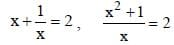⇒ x2 + 1 = 2x
⇒ x2 – 2x + 1 = (x – 1)2
∴ x – 1 = 0 ∴ x = 1
∴ x64 + x121 = 1 + 1 = 2.

SSC CGL Previous Year Questions: Algebra- 2 - Question 14

If x + (1/x) = 4, then what is the value of x6 + (1//x6)?       (SSC CGL 2017)

Detailed Solution for SSC CGL Previous Year Questions: Algebra- 2 - Question 14

x + (1/x)= 4
then,
x6 + (1/x6) = ?
Let x + (1/x) = a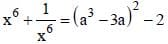⇒ ((4)3 – 3 × 4 )2 – 2
⇒ (64 – 12)2 – 2 = (2704 – 2) = 2702

SSC CGL Previous Year Questions: Algebra- 2 - Question 15

If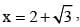then what is the value of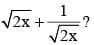(SSC CGL 2017)

Detailed Solution for SSC CGL Previous Year Questions: Algebra- 2 - Question 15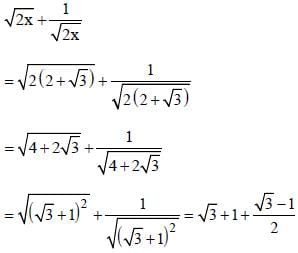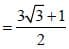SSC CGL Previous Year Questions: Algebra- 2 - Question 16

If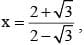then what is the value of x + (1/x)?      (SSC CGL 2017)

Detailed Solution for SSC CGL Previous Year Questions: Algebra- 2 - Question 16

Here,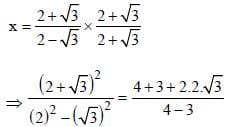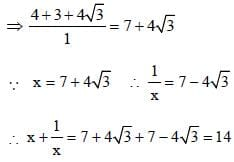SSC CGL Previous Year Questions: Algebra- 2 - Question 17

If 3x + y = 81 and 81x - y = 3, then the value of x/y is       (SSC Sub. Inspector 2016)

Detailed Solution for SSC CGL Previous Year Questions: Algebra- 2 - Question 17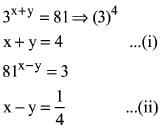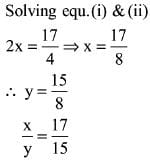SSC CGL Previous Year Questions: Algebra- 2 - Question 18

If p, q, r are all real numbers, then (p – q)3 + (q – r)3 + (r – p)3 is equal to       (SSC Sub. Inspector 2016)

Detailed Solution for SSC CGL Previous Year Questions: Algebra- 2 - Question 18

If a + b + c = 0 then a3 + b3 + c3 = 3abc
let, a = (p – q), b = (q – r), c - (r – p)
than, a + b + c = p – q + q – r + r – p = 0
∴ (p – q)3 + (q – r)2 + (r – p)3
= 3(p – q) (q – r) (r – p) = 0

SSC CGL Previous Year Questions: Algebra- 2 - Question 19

If x = 31/3 – 3–1/3 then 3x3 + 9x is equal to       (SSC CGL 1st Sit. 2016)

Detailed Solution for SSC CGL Previous Year Questions: Algebra- 2 - Question 19

x = 31/3 – 3–1/3
Cubing on both sides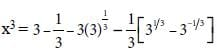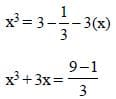3x3 + 9x = 8

SSC CGL Previous Year Questions: Algebra- 2 - Question 20

If x = 222, y = 223, z = 225 then the value of x3 + y3 + z3 – 3xyz is          (SSC CGL 1st Sit. 2016)

Detailed Solution for SSC CGL Previous Year Questions: Algebra- 2 - Question 20

x3 + y3 + z3 – 3xyz = 1/2(x + y + z)
[(x – y)2 + (y – z)2 + (z – x)2]
= 1/2(222 + 223 + 225)
[(222 – 223)2 + (223 – 225)2 + (225 – 222)2]
⇒ 1/2[1+4+9] = 335  = 4690

SSC CGL Previous Year Questions: Algebra- 2 - Question 21

If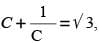then the value of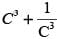is equal to       (SSC CGL 1st Sit. 2016)

Detailed Solution for SSC CGL Previous Year Questions: Algebra- 2 - Question 21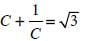Cubing both Sides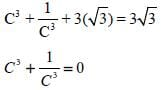SSC CGL Previous Year Questions: Algebra- 2 - Question 22

If 4x + (1/x) = 5, x ≠ 0, then the value of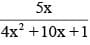is       (SSC CGL 1st Sit. 2016)

Detailed Solution for SSC CGL Previous Year Questions: Algebra- 2 - Question 22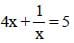⇒ 4x2 + 1 = 5x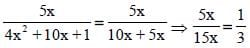SSC CGL Previous Year Questions: Algebra- 2 - Question 23

If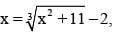then the value of x3 + 5x2 + 12x is       (SSC CGL  1st Sit. 2016)

Detailed Solution for SSC CGL Previous Year Questions: Algebra- 2 - Question 23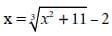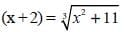(Cubing both sides)
(x + 2)3 = x2 + 11
x3 + 8 + 6x2 + 12x = x2 + 11
x3 + 5x2 + 12x = 3

SSC CGL Previous Year Questions: Algebra- 2 - Question 24

If x =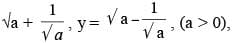then the value of x4 + y4 – 2x2y2 is       (SSC CGL 1st Sit. 2016)

Detailed Solution for SSC CGL Previous Year Questions: Algebra- 2 - Question 24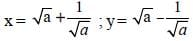x4 + y4 – 2 x2y2
= (x2 – y2)2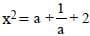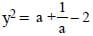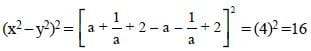SSC CGL Previous Year Questions: Algebra- 2 - Question 25

When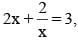then value of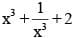is        (SSC CGL  1st Sit. 2016)

Detailed Solution for SSC CGL Previous Year Questions: Algebra- 2 - Question 25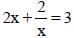Dividing eq by 2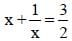Cubing both sides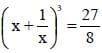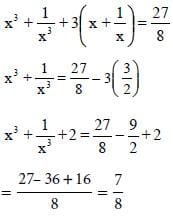SSC CGL Previous Year Questions: Algebra- 2 - Question 26

If (x – 2) (x – p) = x2 – ax + b, then the value of (a – p) is        (SSC CGL 1st Sit. 2016)

Detailed Solution for SSC CGL Previous Year Questions: Algebra- 2 - Question 26

(x – 2) (x – p) = x2 – ax + b
x2 + ( – 2 – p)x + (– P) (–2) = x2 – ax + b
– (2 + p) = – a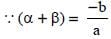2 = a – p

SSC CGL Previous Year Questions: Algebra- 2 - Question 27

If x + y + z = 1,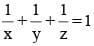and xyz = – 1, then x3 + y3 + zis equal to       (SSC CGL  1st Sit. 2016)

Detailed Solution for SSC CGL Previous Year Questions: Algebra- 2 - Question 27

x + y + z = 1,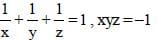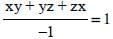xy + yz + zx = – 1
(x + y + z)2 = 1
x2 + y2 + z2 + 2xy + 2yz + 2zx = 1
x2 + y2 +z2 + 2(–1) = 1
x2 + y2 +z2 = 3
x3 + y3 + z3 – 3xyz
= (x + y + z) (x2 + y2 +z2 + xy – yz – zx)
x3 + y3 + z3 – 3(–1) = (1) [(3 – (–1)]
x3 + y3 + z3 + 3 = 4
= 4 – 3 = 1

SSC CGL Previous Year Questions: Algebra- 2 - Question 28

If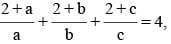then the value of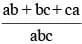is       (SSC CGL   1st Sit. 2016)

Detailed Solution for SSC CGL Previous Year Questions: Algebra- 2 - Question 28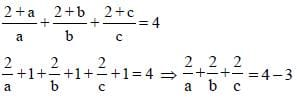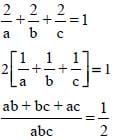SSC CGL Previous Year Questions: Algebra- 2 - Question 29

The length of the base of an isosceles triangle is 2x – 2y + 4z, and its perimeter is 4x – 2y + 6z. Then the length of each of the equal sides is        (SSC CGL  1st Sit. 2016)

Detailed Solution for SSC CGL Previous Year Questions: Algebra- 2 - Question 29

Base side of isosceles Δ = 2x – 2y + 4z
Perimeter = 4x – 2y + 6z
Remaining two sides are = (P-B)/2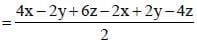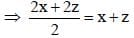SSC CGL Previous Year Questions: Algebra- 2 - Question 30

If x2 – 3x + 1 = 0, (x ≠ 0), then the value of x + (1/x) is       (SSC CGL 1st Sit. 2016)

Detailed Solution for SSC CGL Previous Year Questions: Algebra- 2 - Question 30

x2 – 3x + 1 = 0
Dividing Equation by x
x - 3 + (1/x) = 0
x + (1/x) = 3

SSC CGL Previous Year Questions: Algebra- 2 - Question 31

If  a3 – b3 = 56 and a – b = 2 then what is the value of a2 + b2?       (SSC CGL 1st Sit. 2016)

Detailed Solution for SSC CGL Previous Year Questions: Algebra- 2 - Question 31

(a – b) = 2, a3 – b3 = 56
(a – b)3 = a3 – b3 – 3ab (a – b)
8 = 56 – 3ab (2)
– 48 = – 6ab
∴ ab = 8
(a – b)2 = a2 + b2 – 2ab
4 = a2 + b2 – 16
20 = a2 + b2

SSC CGL Previous Year Questions: Algebra- 2 - Question 32

If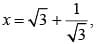then the value of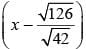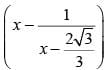is       (SSC CGL 1st Sit. 2016)

Detailed Solution for SSC CGL Previous Year Questions: Algebra- 2 - Question 32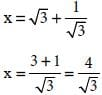Now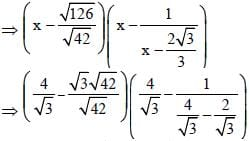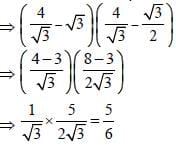SSC CGL Previous Year Questions: Algebra- 2 - Question 33

If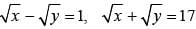then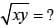(SSC CGL 1st Sit. 2016)

Detailed Solution for SSC CGL Previous Year Questions: Algebra- 2 - Question 33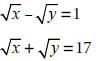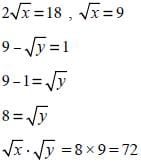SSC CGL Previous Year Questions: Algebra- 2 - Question 34

If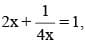then the value of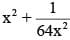is       (SSC CGL  1st Sit. 2016)

Detailed Solution for SSC CGL Previous Year Questions: Algebra- 2 - Question 34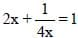dividing Eq. by 2Squaring on both sides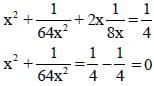SSC CGL Previous Year Questions: Algebra- 2 - Question 35

If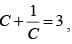then the value of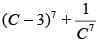is       (SSC CGL 1st Sit. 2016)

Detailed Solution for SSC CGL Previous Year Questions: Algebra- 2 - Question 35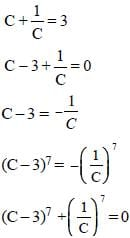SSC CGL Previous Year Questions: Algebra- 2 - Question 36

If x = 2 then the value of x3 + 27x2 + 243x + 631        (SSC CGL 1st Sit. 2015)

Detailed Solution for SSC CGL Previous Year Questions: Algebra- 2 - Question 36

Given x = 2
then x3 + 27x2 + 243x + 631
= 8 + 108 + 486 + 631
= 1233

SSC CGL Previous Year Questions: Algebra- 2 - Question 37

If p = 99 then the value of p (p2 + 3p + 3)        (SSC CGL 1st Sit. 2015)

Detailed Solution for SSC CGL Previous Year Questions: Algebra- 2 - Question 37

Given p = 99
then p(p2 + 3p + 3)
= p((p + 1)2 + p + 2)
= 99 ((99 + 1)2 + 101)
= 99 × (10000 + 101)
= 999999

SSC CGL Previous Year Questions: Algebra- 2 - Question 38

If 5x + 9y = 5 and 125x3 + 729y3 = 120 then the value of the product of x and y is        (SSC CGL 1st Sit. 2015)

Detailed Solution for SSC CGL Previous Year Questions: Algebra- 2 - Question 38

5x +9y = 5 ...(i)
125x3 + 729y3 =120 ...(ii)
Now cube both sides of equation (i), we get
⇒ (5x + 9y)3 = (5)3
⇒ 125x3 + 729y3 +135xy(5x + 9y) =125
⇒ 125x3 + 729y3 +135xy x 5 =125
Now, put the value of equation (i)
120 + 135 xy × 5 = 125
⇒ 135 × 5xy = 125 – 120
⇒ 135 × 5xy = 5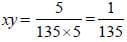SSC CGL Previous Year Questions: Algebra- 2 - Question 39

If x =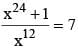then the value of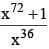(SSC CGL 1st Sit. 2015)

Detailed Solution for SSC CGL Previous Year Questions: Algebra- 2 - Question 39

Given that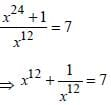On cubing both sides we get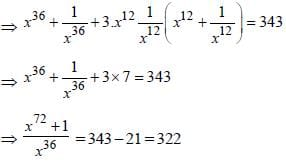SSC CGL Previous Year Questions: Algebra- 2 - Question 40

The HCF of x8 – 1 and x4 + 2x3 – 2x – 1 is:        (SSC CGL 1st Sit. 2015)

Detailed Solution for SSC CGL Previous Year Questions: Algebra- 2 - Question 40

Given fraction
x8 -1 = (x4 +1)(x2 +1)(x -1)(x +1)
x4 + 2x3 - 2x -1 = (x -1)(x +1)3
HCF of Given fractor
= (x -1)(x +1) = x2 -1

## SSC CGL (Tier - 1) - Previous Year Papers (Topic Wise)

250 tests
Information about SSC CGL Previous Year Questions: Algebra- 2 Page
In this test you can find the Exam questions for SSC CGL Previous Year Questions: Algebra- 2 solved & explained in the simplest way possible. Besides giving Questions and answers for SSC CGL Previous Year Questions: Algebra- 2, EduRev gives you an ample number of Online tests for practice

250 tests